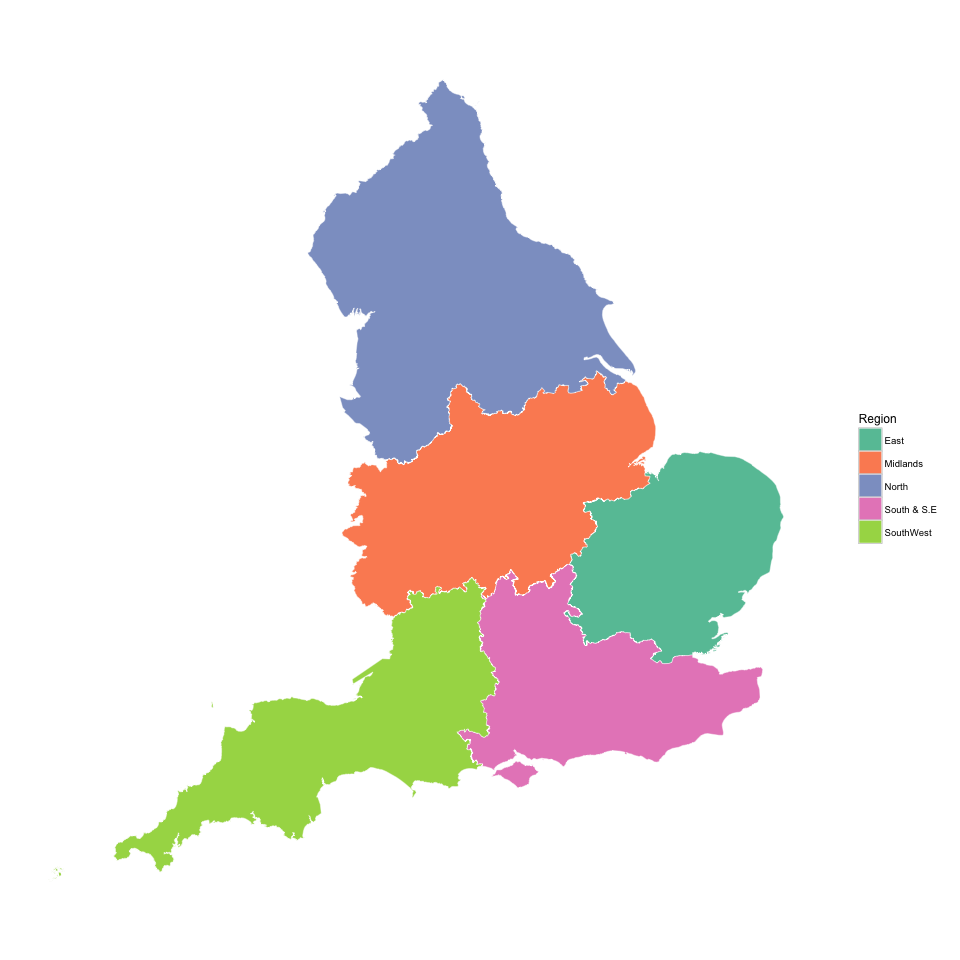rud.is

### Overview

This example comes from a question on the R-Sig-Geo mailing list. This is a small-ish shapefile of England, but the polygons are pretty complex. The inquisitor wants to combine some regions and use the shape with ggplot2.

Let’s get the library calls out of the way:

library(sp)
library(maptools)
library(rgeos)
library(rgdal)
library(dplyr)
library(ggplot2)
library(ggthemes)
library(ggalt)

This bit downloads (without caching) the shapefile and reads it into a SpatialPolygonsDataFrame. Note verbose=FALSE and stringsAsFactors=FALSE on the call to readOGR.

URL <- "http://census.edina.ac.uk/ukborders/easy_download/prebuilt/shape/England_gor_2011.zip"
fil <- basename(URL)

fils <- unzip(fil)
shp <- grep("shp$", fils, value=TRUE) region <- readOGR(shp, ogrListLayers(shp), verbose=FALSE, stringsAsFactors=FALSE) ### Making new regions The person who posted the question wanted to create 5 regions from the 8 regions in the shapefile. We’ll add a column to the data slot with the places where the individual polygon groups belong. region@data$Region <- c("East", "South & S.E", "North", "North", "Midlands",
"North", "SouthWest", "Midlands", "South & S.E")
colnames(region@data) <- c("code", "name","Region")

Now, we need to merge them but we really need to simplify them. Even base plotting takes a while with un-simplified polygons. However, when we do the simplification, it causes topology errors (that aren’t an issue in some GIS systems, but they are in R). So, after we do the merge/dissolve and simplifications, we use rgeos::gBuffer to try to (and successfully) fix the errors.

regions <- unionSpatialPolygons(region, region@data$Region) regions <- gSimplify(regions, 100, topologyPreserve=TRUE) regions <- gBuffer(regions, byid=TRUE, width=0) ### Making pretty maps To use this more effectively with ggplot2 we need to turn it back into a SpatialPolygonsDataFrame. But, we also want to draw the regions well (i.e. with an appropraite projection) and both coord_map and ggalt::coord_proj are not (by default) enamored with the projection the shapefile came with. So, we change it back to simple lat/lon and add in our new region names. regions <- SpatialPolygonsDataFrame(spTransform(regions, CRS("+proj=longlat +ellps=sphere +no_defs")), data.frame(Region=names(regions), row.names=names(regions), stringsAsFactors=FALSE)) Now, we can fortify the SPDF using the new Region names and setup a good projection for the map. eng_map <- fortify(regions, region="Region") eng_proj <- "+proj=aea +lat_0=54.55635146083455 +lon_0=-3.076171875" Finally, we take a look at all our hard work. ggplot() + geom_map(data=eng_map, map=eng_map, aes(x=long, y=lat, map_id=id), color=NA, fill=NA, size=0.5) + geom_map(data=data.frame(id=unique(eng_map$id)), map=eng_map,
aes(map_id=id, fill=id),
color="white", size=0.15) +
scale_fill_brewer(name="Region", palette="Set2") +
coord_proj(eng_proj) +
theme_map() +
theme(legend.position="right")`